#### The "Essential" Excel Formulas for Estimating Concrete, Framing Lumber, Brick, Roofing and Attic Ventilation

This software requires Microsoft Excel 2010 or later.

### Cubic Yard Formulas

You can easily estimate the volume of fill and concrete for:
✔  Slabs and Flatwork
✔  Round Piers
✔  Foundation Beams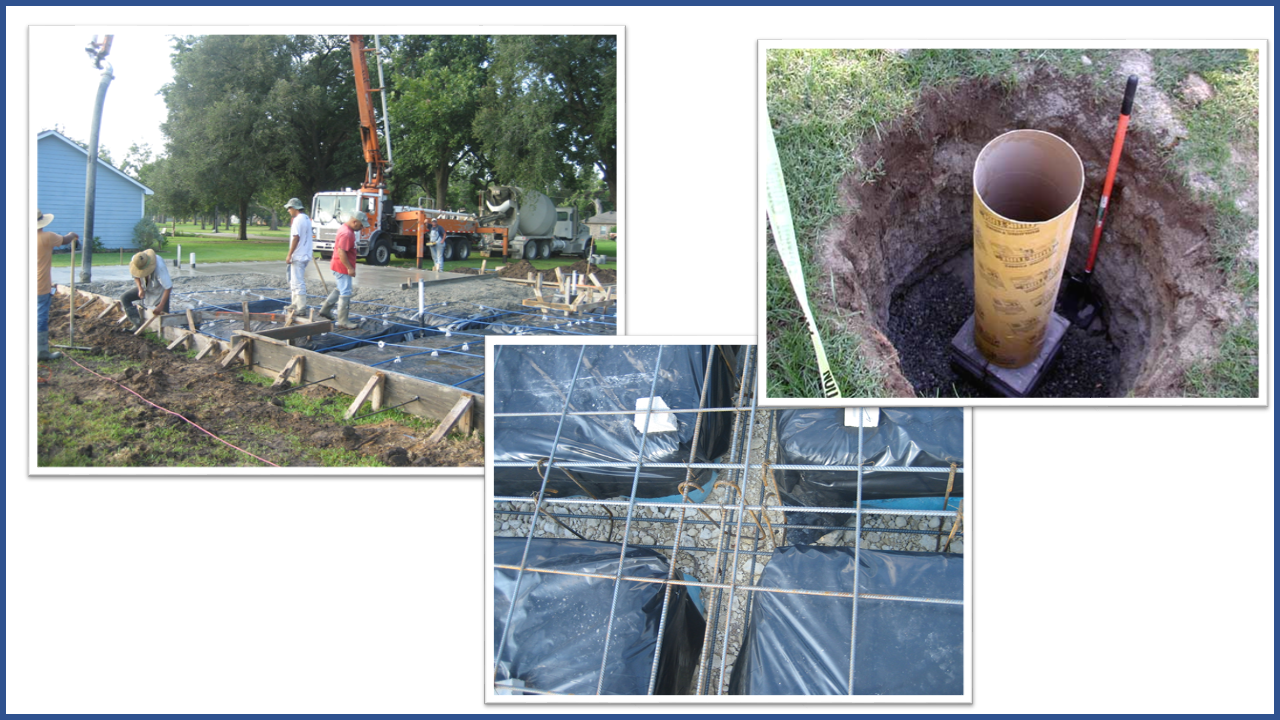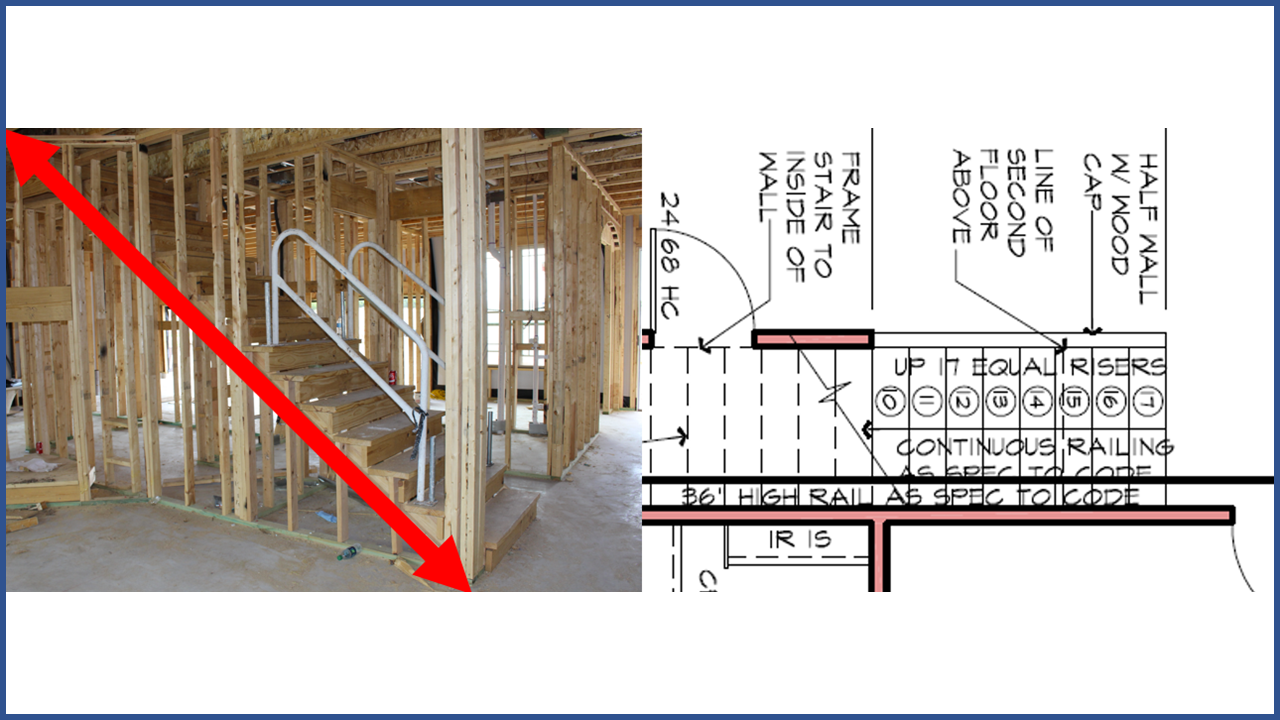### Framing Lumber Takeoff Formulas

Do you ever need to calculate the sloped length of framing members? Use these formulas:
✔  Common Rafters & Barge Rafters
✔  Hip & Valley Rafters (both Equal and Unequal Roof Slopes)
✔  Stair Carriages (and Stair Riser Heights)

### Brick Takeoff Formulas

If you do brick takeoffs, you've got to have these:
✔  Layup Factors (Bricks per Square Foot)
✔  Brick Wall Coverage Areas per Cube
✔  Brick Count and Full Cube Order Quantities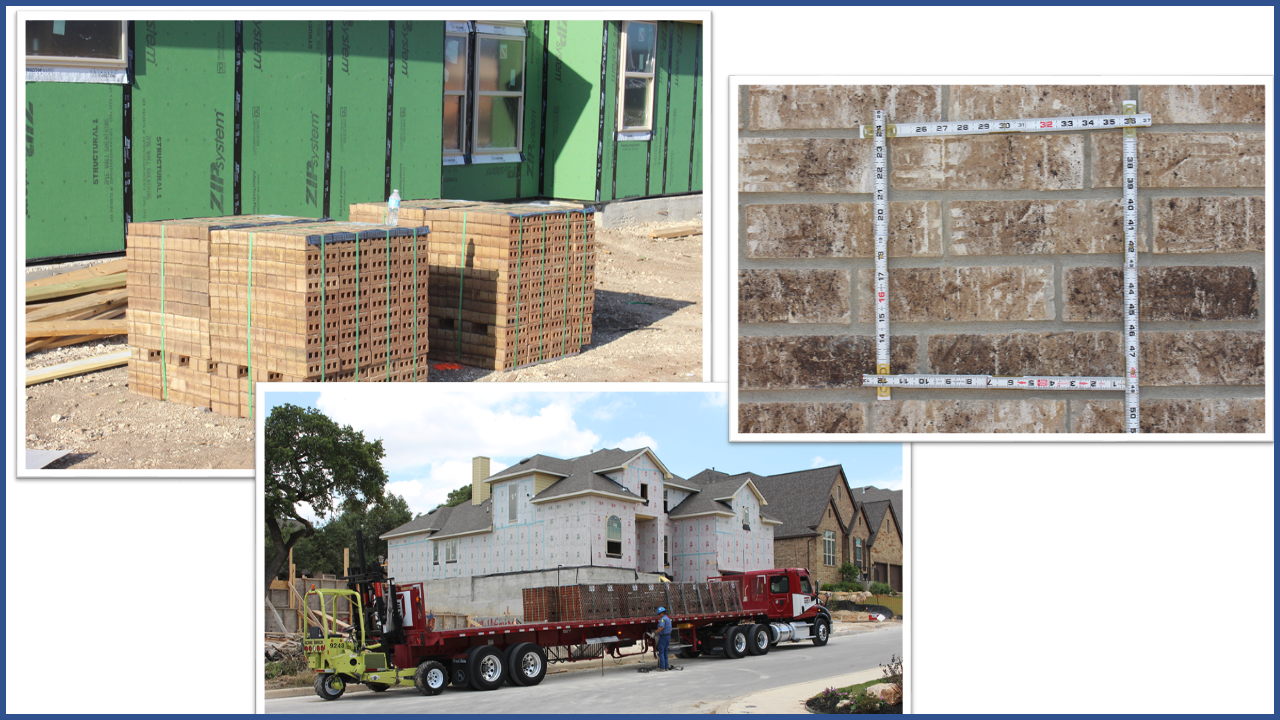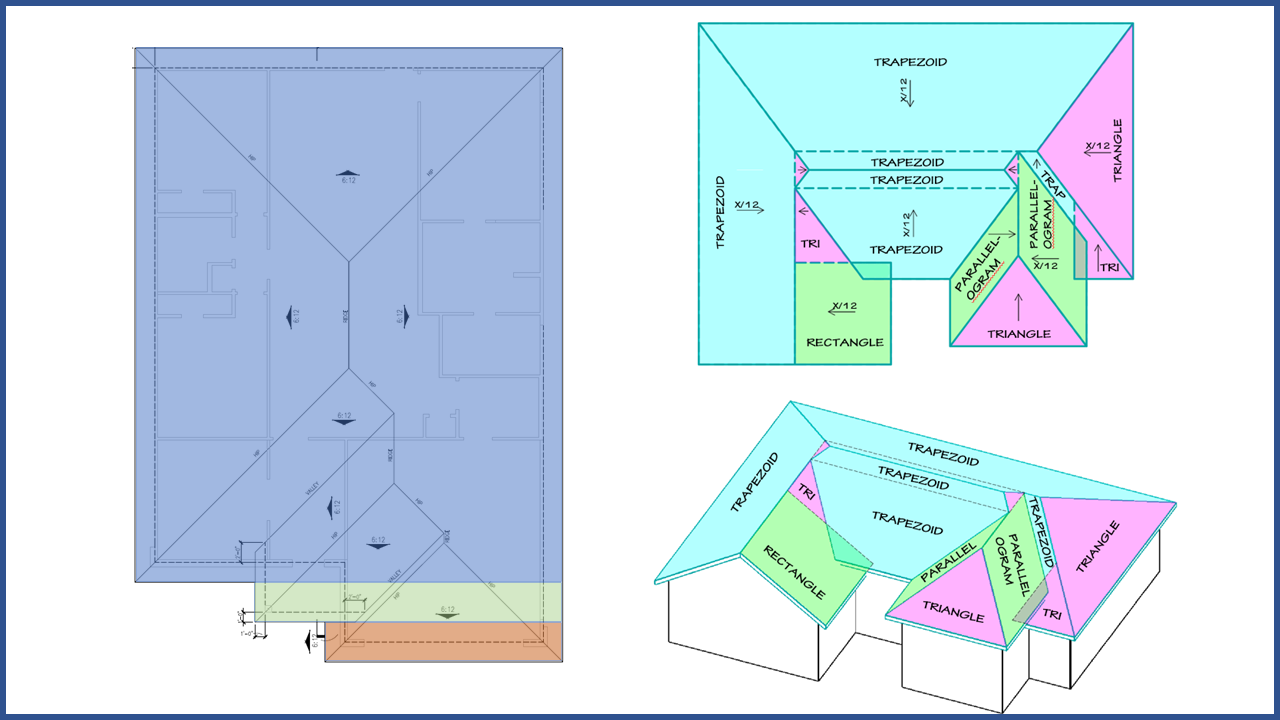### Roofing Takeoff Formulas

These are "must-haves" for roofing and roof decking estimators:
✔  Roof Slope Factors
✔  Roof Surface Area
✔  Squares and Bundles of Shingles

### Roof Ventilation Formulas

Have you evert tried to interpret the Build Code? These make your calculations a breeze:
✔  1/150 Net Free Attic Vent Area
✔  1/300 Net Free Attic Vent Area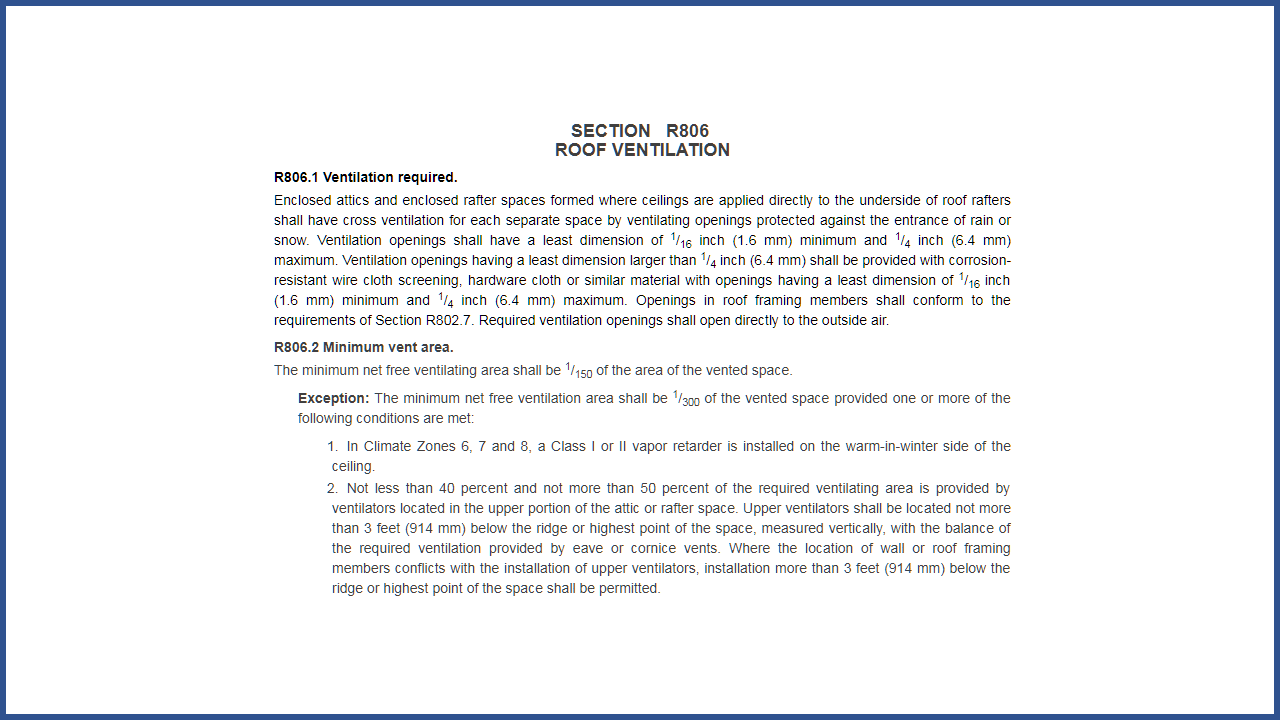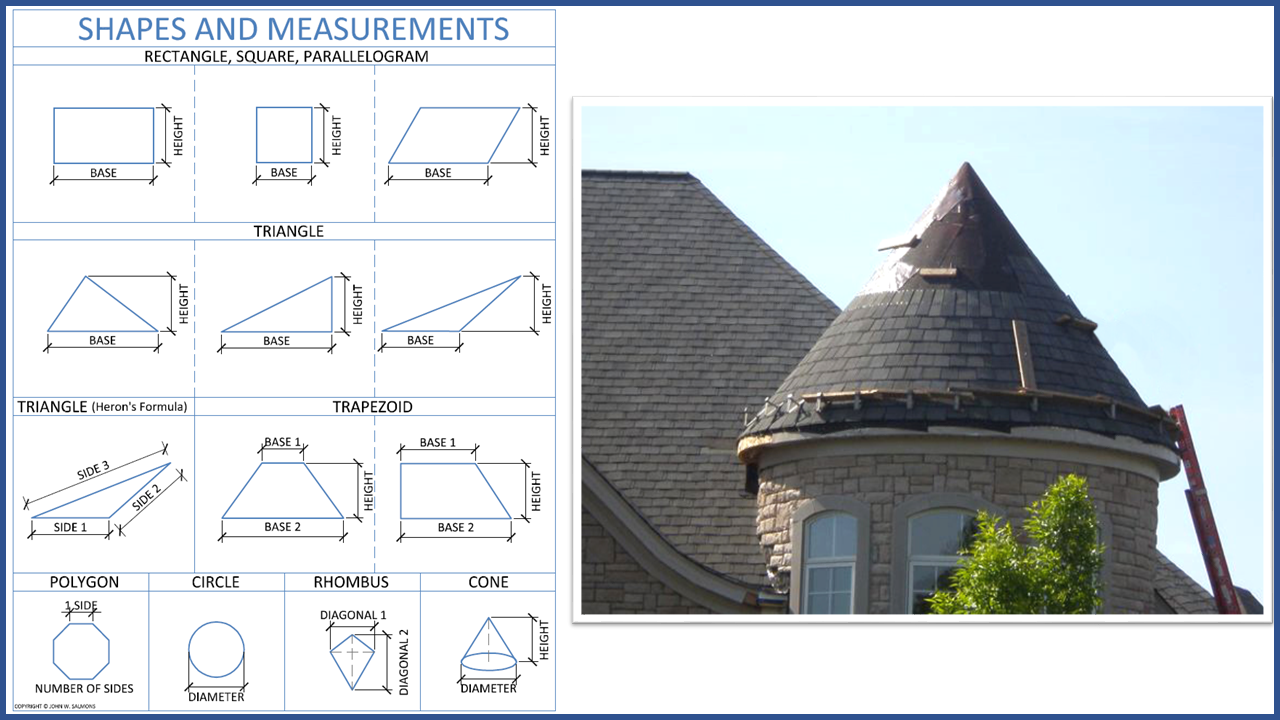### Area Square Footage Formulas

You can easily calculate the area of complex shapes when you're estimating foundations, walls, roofs, topsoil, building sites...
Rectangles, Squares and Parallelograms
✔  Trapezoids
✔  Triangles (including Heron's Formula)
✔  Regular Polygons
✔  Cones
✔  Circles
✔  Rhombuses (Kites)

### Feet-Inches Calculators

Skip the hand-held calculators and use Excel to keep a running total of all your plan measurements.
✔  Feet-Inches-Fractions
✔  Feet-Inches-Sixteenths (F-I-S)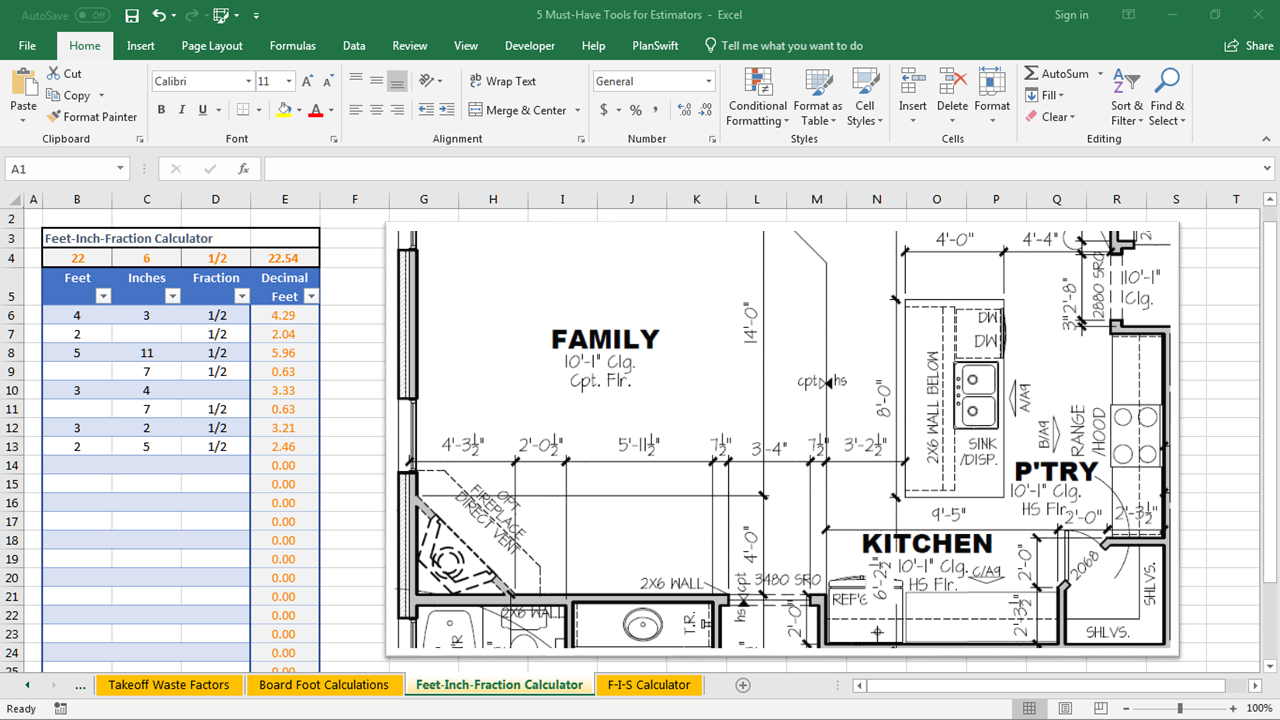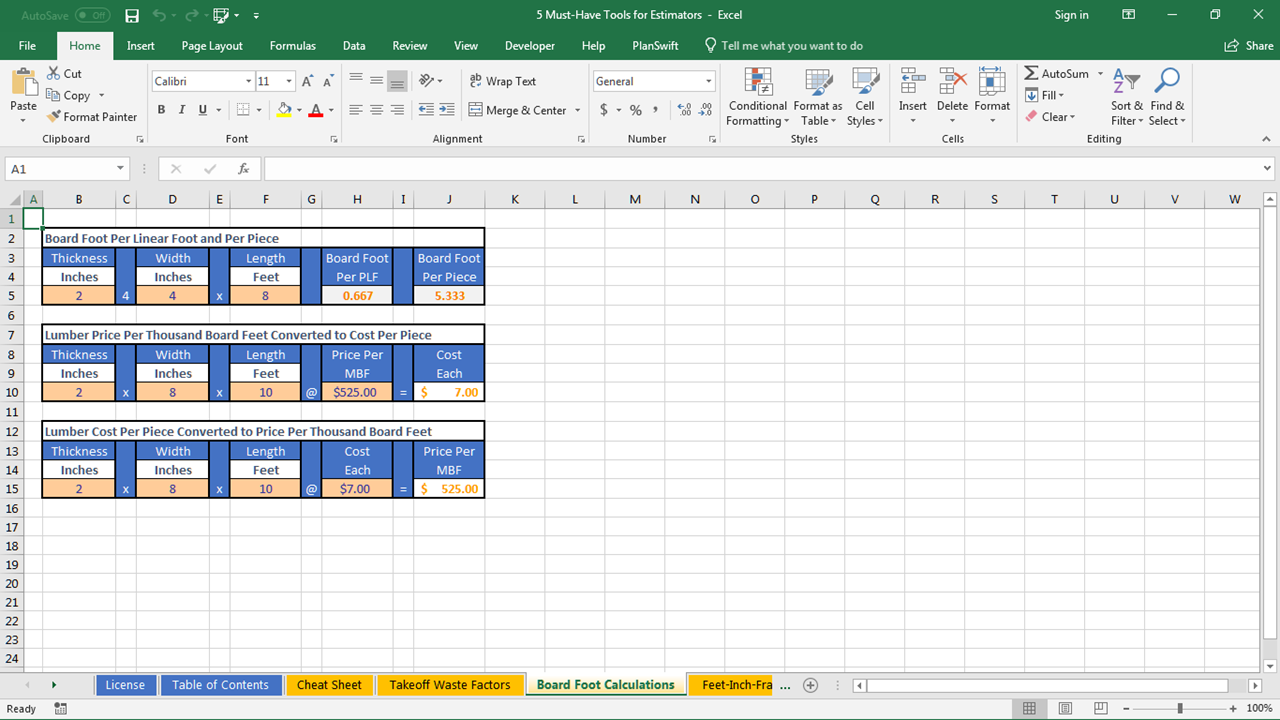### Board Foot Calculators

Do you ever work with Board Feet? These formulas cuts through the confusion:
✔  Board Feet per Linear Foot
✔  Board Feet per Linear Piece
✔  Convert Price Per Thousand to Cost Per Piece
✔  Convert Cost Per Piece to Price Per Thousand

### Estimator's Cheat Sheet

Improve your productivity with these handy references:
✔  Foot/Inch Decimal Equivalents
✔  16" On Center & Foot/Inch Equivalents
✔  Roof Slope Factors
✔  Square Footage Yield per Cubic Yard of Concrete, Fill or Topsoil.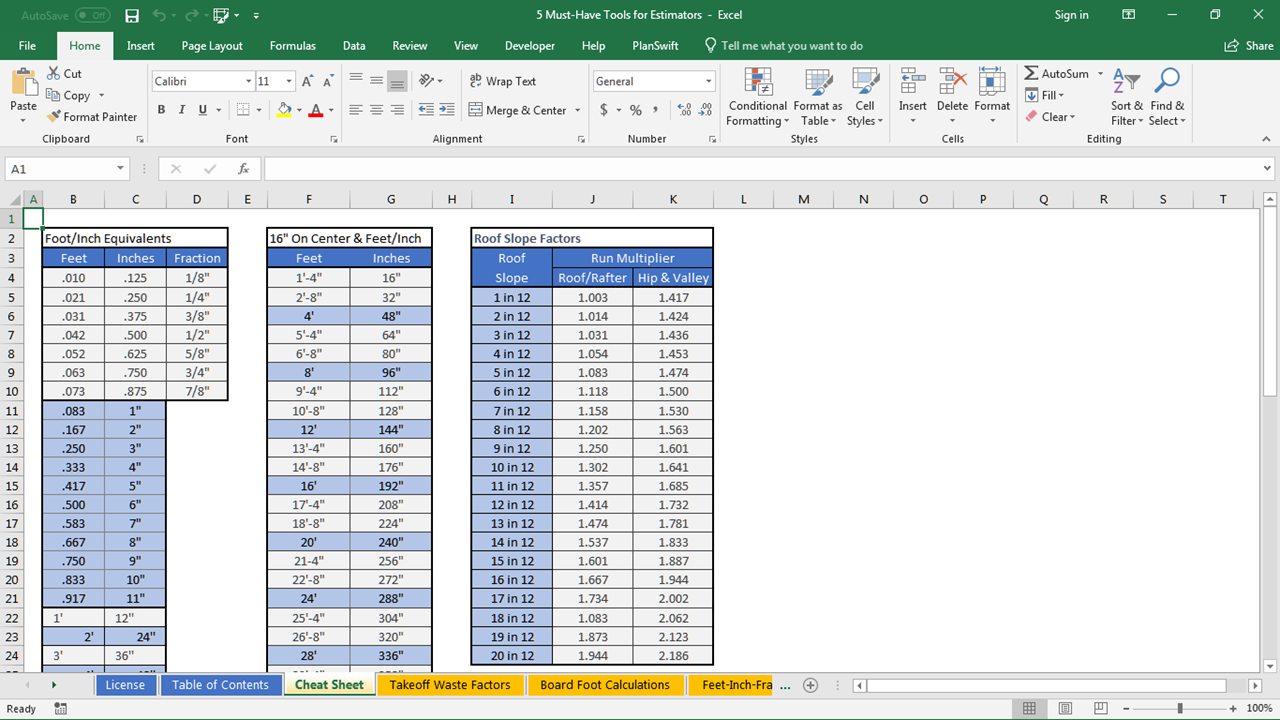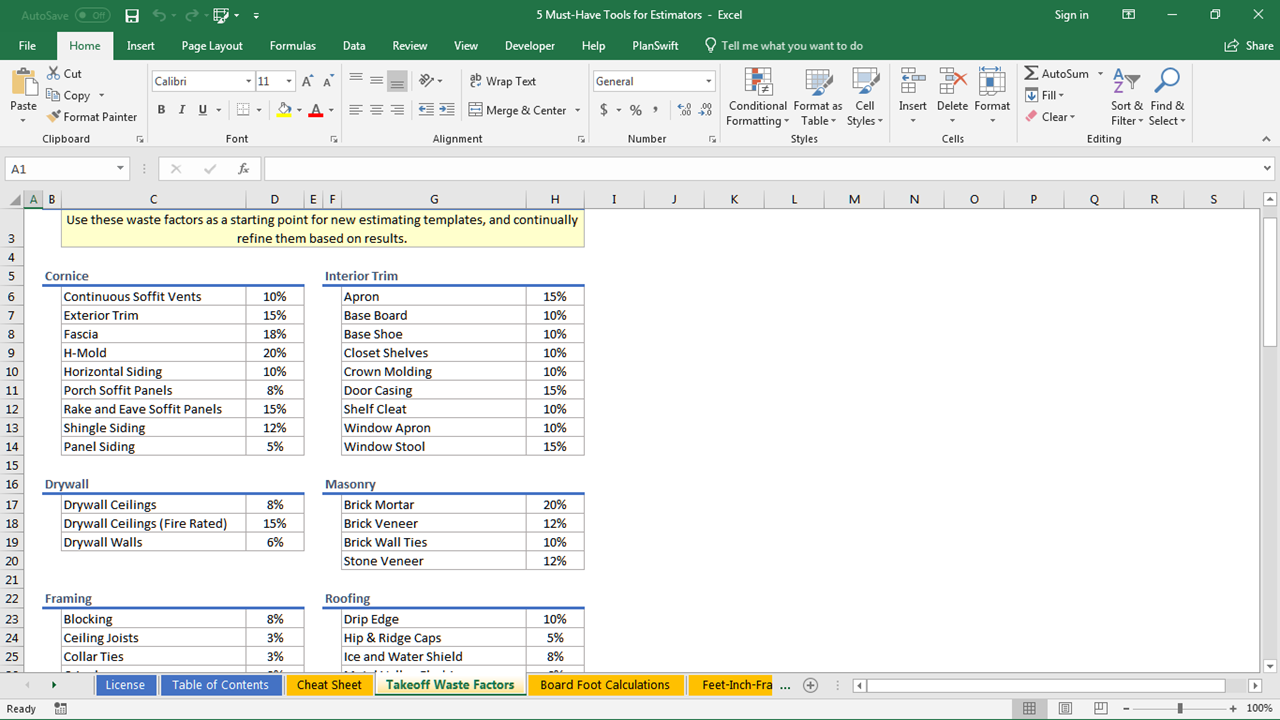### Takeoff Waste Factors

This report lists “field-tested” estimating waste factors for 63 takeoff items - including cornice, drywall, framing interior trim, masonry, roofing, sheathing & decking, and weatherproofing.

As estimators, we know over-estimating or under-estimating can cost thousands. I’ve been refining and using these waste factors over 25 years in the biz – giving trades “just enough” material to finish the job.

This software requires Microsoft Excel 2010 or later.

Save the hassle of writing and testing complex formulas. Use this Excel Workbook as-is... or simply copy and paste these proven Microsoft Excel formulas into your own Estimating Template.

### Video Transcript

Hey there estimators!

Here’s a real quick video on something I call the 25 “Essential” Excel formulas for Estimating. These include formulas for estimating concrete, framing lumber, brick, roofing and roof ventilation. Also, some very simple formulas for calculating the square footage complex shapes.

If you don’t know me - my name is John Salmons. For over 25 years, I’ve been doing material takeoffs for many of the largest (and also some of the smallest) homebuilders in the US. And along the way, I’ve written a ton of Excel formulas for Estimating just about every building material there is.

And what I’m about to show you is my short list of the 25 “essentials” … all bundled into one power-packed Excel workbook.

The first set of formulas I’d like to show is a fast and accurate way to calculate the sloped length of hip & valley rafters from roof plans. All you need to do is measure the hip or valley line and enter it - and the roof slopes into these formulas. This works with equal and multi-pitched roofs, and it’s also a great formula to estimate roofing hip & ridge caps.

And here’s a quick tip: For every 31 linear feet of hip & ridge – order one bundle of three-tab shingles.

Speaking of roofing - Do you want to know a simple and accurate way to measure even the most complex roofs You can break just about any roof into rectangles, triangles and trapezoids. From there -  all you’ll need to do is enter the roof slope and the measurements into these formulas - giving you the roofing square footage, squares and bundles of shingles. Or, if you already know the square footage, you can enter that - plus the roof slope into this formula… to calculate the sloped square footage, and the number of squares and bundles of roofing shingles.

And, you can use this formula to calculate the roof slope factor for any roof pitch… its essential for estimating roof decking and shingles, from roof plans.

Do you need to estimate concrete volume for slabs, foundation beams, and round piers? You can use these concrete formulas to calculate cubic yard of concrete.

How about calculating the length of 2x12 stair stringers, or the horizontal run of the stairs, or even the height of your stair risers (making sure you’re not exceeding the building code’s 7 ¾” max. height)? You can do all three… using this one formula. It’s also a great way to estimate handrail, too.

Would you like to know the square footage of brick veneer wall area packed into each cube of brick – or how many cubes to order? How about calculating the number of bricks per-square-foot of wall area? This is known as the layup factor. These three brick formulas do exactly that for you.

Ever try to interpret the building code for proper roof ventilation? Use these formulas for calculating the size and number of roof and soffit vents – giving you a well-balanced system.

Do you need to calculate the square footage of any of these shapes? Or even - roofing shingles on a tower? Just enter a few basic measurements into these formulas to get the exact square footage.

Here’s one I particularly like for calculating the square footage of obtuse triangles – just by measuring the three sides. It’s called Heron’s Formula - and it really comes in handy when measuring triangular areas… especially on step roofs!

Finally, if you need to calculate the sloped length of common rafters or barge rafters from roof plans…use this formula. You can also use it to estimate roofing starter strip along the rakes. Just figure one bundle of three-tab shingles for every 75 linear feet.

That’s all 25 “Essential” Formulas for Estimating… but I have a few more things estimators need. I call this the 5 “Must Have” Tools for Estimators.

First is my “Cheat Sheet”.  This, I always keep at arm’s reach. It’s a one-pager that includes Foot/Inch/Decimal equivalents, 16” on center spacing listed in feet and inches, common roof slope factors, and the square foot coverage per cubic yard - great when estimating concrete, topsoil and fill.

Next are 63 “field-tested” waste factors for estimating cornice, drywall, framing, interior trim, masonry, roofing, sheathing & decking, and weatherproofing. It’ll be interesting to compare these to what you’re using.

If you happen to work with board-feet and price-per-thousand, you can use these formulas to calculate board feet for any size dimension lumber. And you can easily convert price-per-thousand to cost-each, and cost-each to price-per-thousand.

And finally, the last two “Must-Have” tools for estimators are the Excel Foot/Inch calculators. The first allows you to add and subtract strings of architectural dimension (including fractions) – which is great for laying out the precise locations of kitchen islands, plumbing stacks and light fixtures. And the second is for calculating those “odd-looking” hyphenated dimensions – commonly used on roof truss and wall panel designs. They’re abbreviated as F-I-S… short for Feet-Inches-Sixteenths measurements. And I’ll but you’ll like using them so much - you may never want to use fractions again!